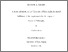# FINITELY ADDITIVE MEASURES ON TOPOLOGICAL SPACES AND BOOLEAN ALGEBRAS

Ameen, Zanyar (2015) FINITELY ADDITIVE MEASURES ON TOPOLOGICAL SPACES AND BOOLEAN ALGEBRAS. Doctoral thesis, University of East Anglia.Preview PDF Download (1MB) | Preview

## Abstract

The thesis studies some problems in measure theory. In particular, a possible generalization
corresponding to Maharam Theorem for �nitely additive measures (charges).
In the �rst Chapter, we give some de�nitions and results on di�erent areas of Mathematics
that will be used during this work.
In Chapter two, we recall the de�nitions of nonatomic, continuous and Darboux
charges, and show their relations tWe hereby con�rm that all work without reference are
our work excluding the chapter one. We hereby con�rm that all work without reference
are our work excluding the chapter one. o each other. The relation between charges
on Boolean algebras and the induced measures on their Stone spaces is mentioned
in this chapter. We also show that for any charge algebra, there exists a compact
zero-dimensional space such that its charge algebra is isomorphic to the given charge
algebra.
In Chapter three, we give the de�nition of Jordan measure and some of its outcomes.
We de�ne another measure on an algebra of subsets of some set called Jordanian measure,
and investigate it. Then we de�ne the Jordan algebras and Jordanian algebras,
and study some of their properties.
Chapter four is mostly devoted to the investigation of uniformly regular measures
and charges (on both Boolean algebras and topological spaces). We show how the
properties of a charge on a Boolean algebra can be transferred to the induced measure
on its Stone space. We give a di�erent proof to a result by Mercourakis in [36, Remark
1.10]. In 2013, Borodulin-Nadzieja and Dºamonja [10, Theorem 4.1] proved the countable
version of Maharam Theorem for charges using uniform regularity. We show that
3
4
this result can be proved under weaker assumption and further extended.
The �nal Chapter is concerned with the higher versions of uniform regularity which
are called uniform �-regularity. We study these types of measures and obtain several
results and characterizations. The major contribution to this work is that we show
we cannot hope for a higher analogue of Maharam Theorem for charges using uniform
�-regularity. In particular, Theorem 4.1 in  and Remark 1.10 in  cannot be
extended for all cardinals. We prove that a higher version of Theorem 4.1 in 
(resp. Remark 1.10 in ) can be proved only for charges on free algebras on � many
generators (resp. measures on a product of compact metric spaces). We also generalize
Proposition 2.10 in .

Item Type: Thesis (Doctoral) Faculty of Science > School of Mathematics Users 2259 not found. 29 Jan 2016 09:33 29 Jan 2016 09:33 https://ueaeprints.uea.ac.uk/id/eprint/56864View Item